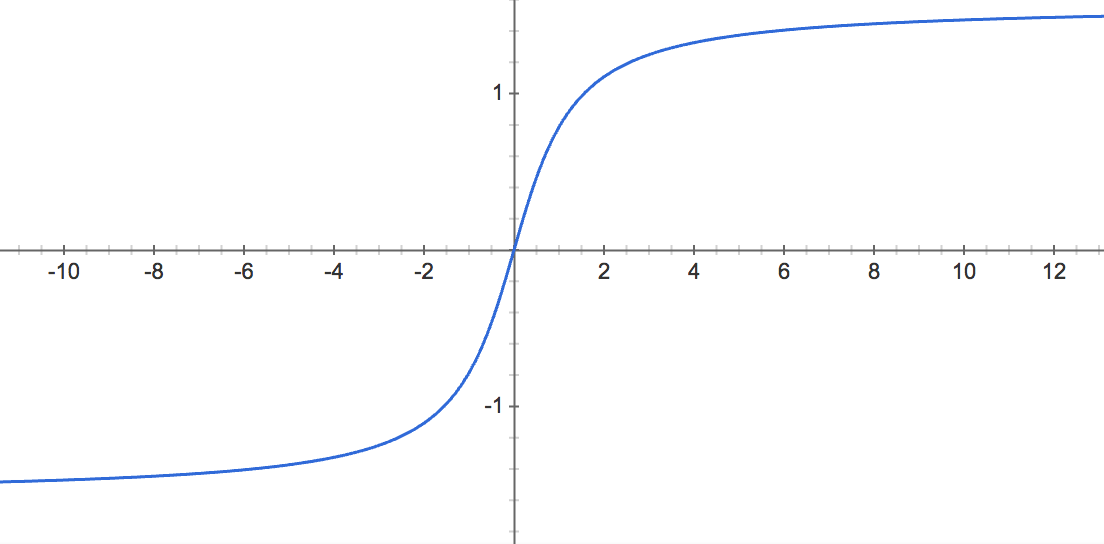# Arctangent function

Arctan(x), tan-1(x), inverse tangent function.

Contents

### Arctan definition

The arctangent of x is defined as the inverse tangent function of x when x is real (x∈ℝ).

When the tangent of y is equal to x:

tan y = x

Then the arctangent of x is equal to the inverse tangent function of x, which is equal to y:

arctan = tan-1 x = y

For example:

arctan 1 = tan-1 1 = π/4 rad = 45°

### f(x) = arctan(x)